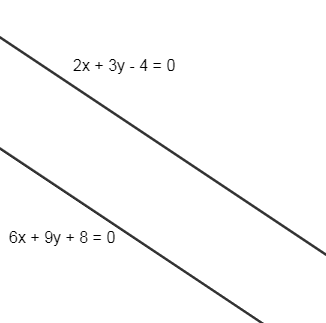Courses
Courses for Kids
Free study material
Free LIVE classes
MoreLIVE
Join Vedantu’s FREE Mastercalss

# Choose the correct statement which describes the position of the point $\left( -6,2 \right)$ relative to straight lines $2x+3y-4=0$ and $6x+9y+8=0$.a)Below both the linesb)Above both the linesc)In between the linesd)None of theseVerified
359.7k+ views
Hint:Use position of a point with relative to a line and check the condition to solve this problem.

The equations of two lines are $2x+3y-4=0$ and $6x+9y+8=0$. It can be represented as shown below.A point $\left( {{x}_{1}},\text{ }{{y}_{1}} \right)$ will lie below the line $ax+by+c=0$ if $a{{x}_{1}}+b{{y}_{1}}+c<0$ and vice versa.
We will find the position of the point with respect to first line, i.e., $2x+3y-4=0$, i.e., substitute $\left( -6,2 \right)$ in the line equation, we get
$2(-6)+3(2)-4$
$-12+6-4$
$-10<0$
So, the given point $\left( -6,2 \right)$ is below the line $2x+3y-4=0$.
Now we will find the position of the point with respect to second line, i.e., $6x+9y+8=0$, i.e., substitute$\left( -6,2 \right)$in the line equation, we get
$6(-6)+9(2)+8$
$-36+18+8$
$-10<0$
So, the given point $\left( -6,2 \right)$is below the line $6x+9y+8=0$.
So, the point $\left( -6,2 \right)$is below both the given lines.
Hence the correct answer is option (a).

Note: The possibility of error is that instead of less than it can be considered greater than, i.e., if the $a{{x}_{1}}+b{{y}_{1}}+c>0$then the point is below the line. In this case we will get the wrong answer.
Last updated date: 22nd Sep 2023
Total views: 359.7k
Views today: 3.59k